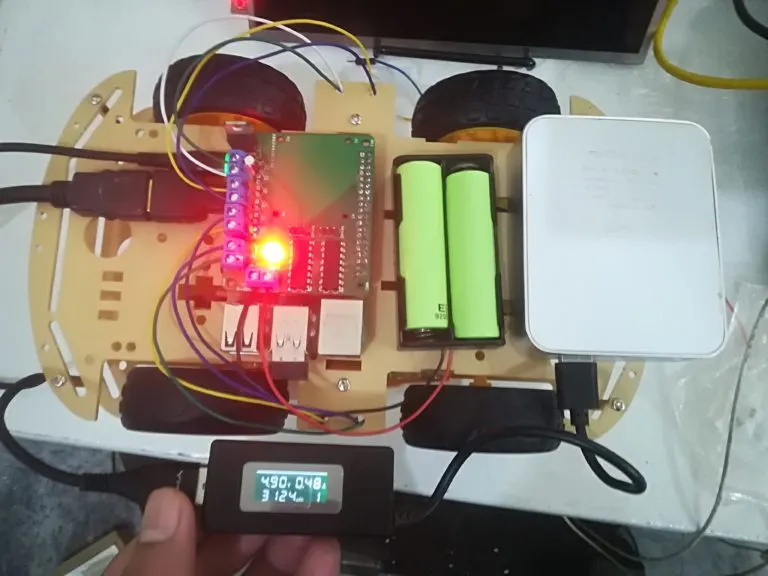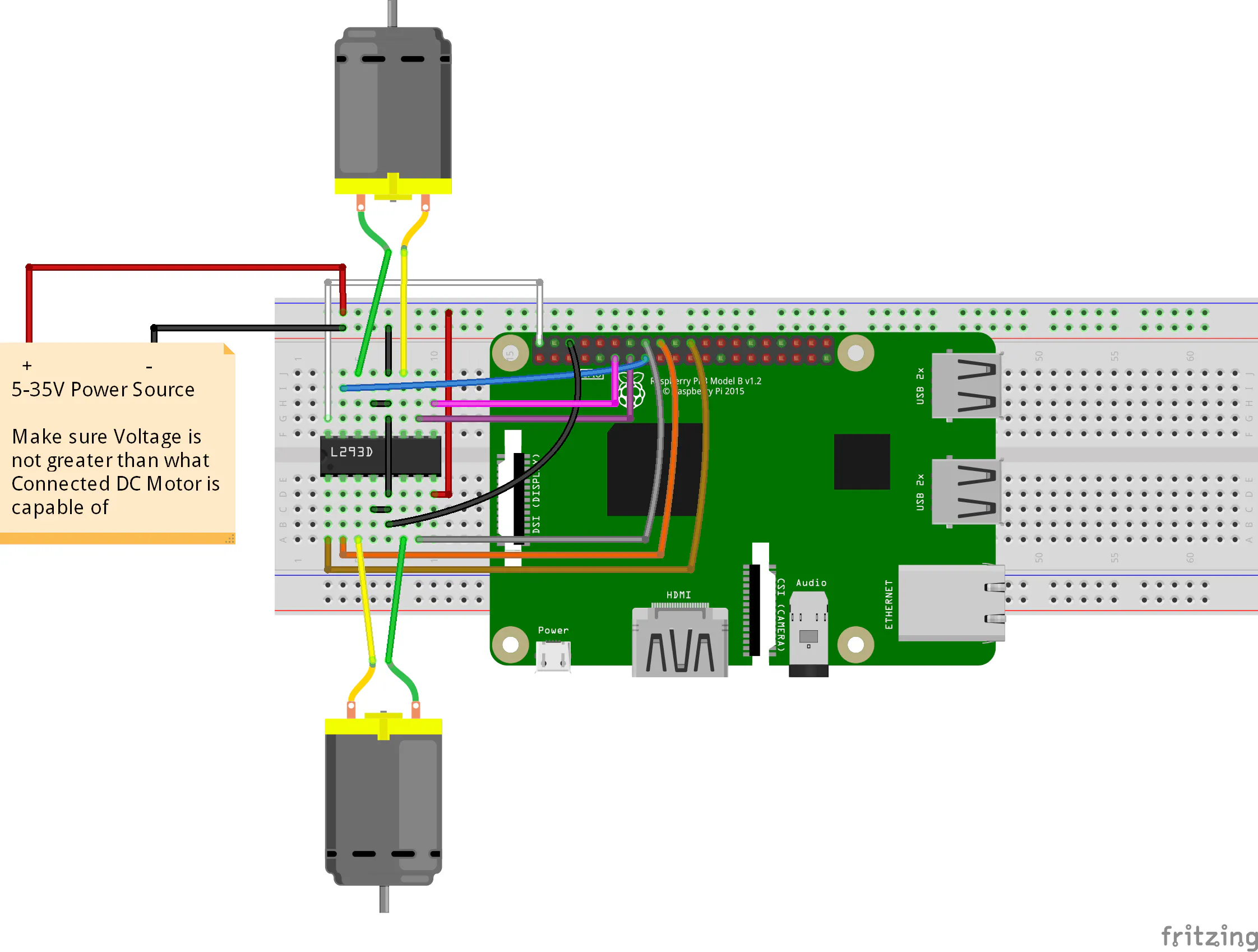Published

# Raspberry Pi DC Motor Control with Custom Board

In this tutorial, you are going to learn about how to control DC motor with Raspberry Pi.

BeginnerProtip2 hours84## Schematics

### raspberry_pi_l293d_bb_iagVujXtqd.png## Code

### Code snippet #1

Plain text
```import RPi.GPIO as GPIO
from time import sleep

GPIO.setmode(GPIO.BCM)
GPIO.setwarnings(False)

Motor1 = {'EN': 25, 'input1': 24, 'input2': 23}
Motor2 = {'EN': 17, 'input1': 27, 'input2': 22}

for x in Motor1:
GPIO.setup(Motor1[x], GPIO.OUT)
GPIO.setup(Motor2[x], GPIO.OUT)

EN1 = GPIO.PWM(Motor1['EN'], 100)
EN2 = GPIO.PWM(Motor2['EN'], 100)

EN1.start(0)
EN2.start(0)

while True:
for x in range(40, 100):
print ("FORWARD MOTION")
EN1.ChangeDutyCycle(x)
EN2.ChangeDutyCycle(x)

GPIO.output(Motor1['input1'], GPIO.HIGH)
GPIO.output(Motor1['input2'], GPIO.LOW)

GPIO.output(Motor2['input1'], GPIO.HIGH)
GPIO.output(Motor2['input2'], GPIO.LOW)

sleep(0.1)

print ("STOP")
EN1.ChangeDutyCycle(0)
EN2.ChangeDutyCycle(0)

sleep(5)

for x in range(40, 100):
print ("BACKWARD MOTION")
EN1.ChangeDutyCycle(x)
EN2.ChangeDutyCycle(x)

GPIO.output(Motor1['input1'], GPIO.LOW)
GPIO.output(Motor1['input2'], GPIO.HIGH)

GPIO.output(Motor2['input1'], GPIO.LOW)
GPIO.output(Motor2['input2'], GPIO.HIGH)

sleep(0.1)

print ("STOP")
EN1.ChangeDutyCycle(0)
EN2.ChangeDutyCycle(0)

sleep(5)

GPIO.cleanup()
```

### Code snippet #2

Plain text
```import RPi.GPIO as GPIO
from time import sleep

GPIO.setmode(GPIO.BCM)
GPIO.setwarnings(False)

Motor1 = {'EN': 21, 'input1': 20, 'input2': 16}
Motor2 = {'EN': 6, 'input1': 19, 'input2': 26}
Motor3 = {'EN': 25, 'input1': 23, 'input2': 24}
Motor4 = {'EN': 17, 'input1': 27, 'input2': 22}

for x in Motor1:
GPIO.setup(Motor1[x], GPIO.OUT)
GPIO.setup(Motor2[x], GPIO.OUT)
GPIO.setup(Motor3[x], GPIO.OUT)
GPIO.setup(Motor4[x], GPIO.OUT)

EN1 = GPIO.PWM(Motor1['EN'], 100)
EN2 = GPIO.PWM(Motor2['EN'], 100)
EN3 = GPIO.PWM(Motor3['EN'], 100)
EN4 = GPIO.PWM(Motor4['EN'], 100)

EN1.start(0)
EN2.start(0)
EN3.start(0)
EN4.start(0)

while True:
for x in range(40, 100):
print ("FORWARD MOTION")
EN1.ChangeDutyCycle(x)
EN2.ChangeDutyCycle(x)
EN3.ChangeDutyCycle(x)
EN4.ChangeDutyCycle(x)

GPIO.output(Motor1['input1'], GPIO.HIGH)
GPIO.output(Motor1['input2'], GPIO.LOW)

GPIO.output(Motor2['input1'], GPIO.HIGH)
GPIO.output(Motor2['input2'], GPIO.LOW)

GPIO.output(Motor3['input1'], GPIO.HIGH)
GPIO.output(Motor3['input2'], GPIO.LOW)

GPIO.output(Motor4['input1'], GPIO.HIGH)
GPIO.output(Motor4['input2'], GPIO.LOW)
sleep(0.1)

print ("STOP")
EN1.ChangeDutyCycle(0)
EN2.ChangeDutyCycle(0)
EN3.ChangeDutyCycle(0)
EN4.ChangeDutyCycle(0)

sleep(5)

for x in range(40, 100):
print ("BACKWARD MOTION")
EN1.ChangeDutyCycle(x)
EN2.ChangeDutyCycle(x)
EN3.ChangeDutyCycle(x)
EN4.ChangeDutyCycle(x)

GPIO.output(Motor1['input1'], GPIO.LOW)
GPIO.output(Motor1['input2'], GPIO.HIGH)

GPIO.output(Motor2['input1'], GPIO.LOW)
GPIO.output(Motor2['input2'], GPIO.HIGH)

GPIO.output(Motor3['input1'], GPIO.LOW)
GPIO.output(Motor3['input2'], GPIO.HIGH)

GPIO.output(Motor4['input1'], GPIO.LOW)
GPIO.output(Motor4['input2'], GPIO.HIGH)
sleep(0.1)

print ("STOP")
EN1.ChangeDutyCycle(0)
EN2.ChangeDutyCycle(0)
EN3.ChangeDutyCycle(0)
EN4.ChangeDutyCycle(0)

sleep(5)

GPIO.cleanup()
```